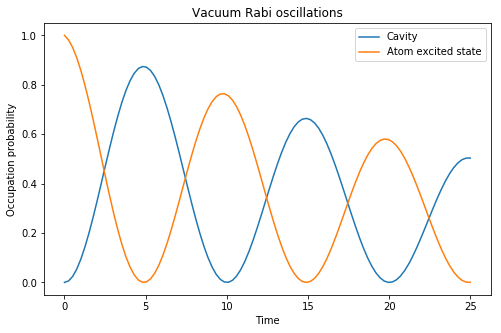# QuTiP example: Vacuum Rabi oscillations in the Jaynes-Cummings model¶

J.R. Johansson and P.D. Nation

This ipython notebook demonstrates how to simulate the quantum vacuum rabi oscillations in the Jaynes-Cumming model, using QuTiP: The Quantum Toolbox in Python.

In :
%matplotlib inline

In :
import matplotlib.pyplot as plt

In :
import numpy as np

In :
from qutip import *


# Introduction¶

The Jaynes-Cumming model is the simplest possible model of quantum mechanical light-matter interaction, describing a single two-level atom interacting with a single electromagnetic cavity mode. The Hamiltonian for this system is (in dipole interaction form)

$H = \hbar \omega_c a^\dagger a + \frac{1}{2}\hbar\omega_a\sigma_z + \hbar g(a^\dagger + a)(\sigma_- + \sigma_+)$

or with the rotating-wave approximation

$H_{\rm RWA} = \hbar \omega_c a^\dagger a + \frac{1}{2}\hbar\omega_a\sigma_z + \hbar g(a^\dagger\sigma_- + a\sigma_+)$

where $\omega_c$ and $\omega_a$ are the frequencies of the cavity and atom, respectively, and $g$ is the interaction strength.

### Problem parameters¶

Here we use units where $\hbar = 1$:

In :
wc = 1.0  * 2 * np.pi  # cavity frequency
wa = 1.0  * 2 * np.pi  # atom frequency
g  = 0.05 * 2 * np.pi  # coupling strength
kappa = 0.005          # cavity dissipation rate
gamma = 0.05           # atom dissipation rate
N = 15                 # number of cavity fock states
n_th_a = 0.0           # temperature in frequency units
use_rwa = True

tlist = np.linspace(0,25,100)


### Setup the operators, the Hamiltonian and initial state¶

In :
# intial state

# operators
a  = tensor(destroy(N), qeye(2))
sm = tensor(qeye(N), destroy(2))

# Hamiltonian
if use_rwa:
H = wc * a.dag() * a + wa * sm.dag() * sm + g * (a.dag() * sm + a * sm.dag())
else:
H = wc * a.dag() * a + wa * sm.dag() * sm + g * (a.dag() + a) * (sm + sm.dag())


### Create a list of collapse operators that describe the dissipation¶

In :
c_op_list = []

rate = kappa * (1 + n_th_a)
if rate > 0.0:
c_op_list.append(np.sqrt(rate) * a)

rate = kappa * n_th_a
if rate > 0.0:
c_op_list.append(np.sqrt(rate) * a.dag())

rate = gamma
if rate > 0.0:
c_op_list.append(np.sqrt(rate) * sm)


### Evolve the system¶

Here we evolve the system with the Lindblad master equation solver, and we request that the expectation values of the operators $a^\dagger a$ and $\sigma_+\sigma_-$ are returned by the solver by passing the list [a.dag()*a, sm.dag()*sm] as the fifth argument to the solver.

In :
output = mesolve(H, psi0, tlist, c_op_list, [a.dag() * a, sm.dag() * sm])


## Visualize the results¶

Here we plot the excitation probabilities of the cavity and the atom (these expectation values were calculated by the mesolve above). We can clearly see how energy is being coherently transferred back and forth between the cavity and the atom.

In :
fig, ax = plt.subplots(figsize=(8,5))
ax.plot(tlist, output.expect, label="Cavity")
ax.plot(tlist, output.expect, label="Atom excited state")
ax.legend()
ax.set_xlabel('Time')
ax.set_ylabel('Occupation probability')
ax.set_title('Vacuum Rabi oscillations');### Software version:¶

In :
from qutip.ipynbtools import version_table

version_table()

Out:
SoftwareVersion
QuTiP4.3.0.dev0+6e5b1d43
Numpy1.13.1
SciPy0.19.1
matplotlib2.0.2
Cython0.25.2
Number of CPUs2
BLAS InfoINTEL MKL
IPython6.1.0
Python3.6.2 |Anaconda custom (x86_64)| (default, Jul 20 2017, 13:14:59) [GCC 4.2.1 Compatible Apple LLVM 6.0 (clang-600.0.57)]
OSposix [darwin]
Thu Jul 20 22:29:51 2017 MDT